## Square Wells p.4

### Finite Square Well

It will be convenient in working with finite square wells to use the symmetric origin choice as symmetry will guarantee that if the solution works at x=a then it must work at x=-a. Furthermore, since the potential is symmetric (and the solutions non-degenerate) the solutions must also either be symmetric (cosine-like or "even") or antisymmetric (sine-like or "odd"). Thus we will have either cos or sin terms, not a linear combination of both. Outside of the well we must have± exp[-x]

for x>a and± exp[+x]

for x<-a as0 as |x|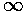to produce normalizable wavefunctions.

Our solutions for |x|<a are:Inside the well the potential is zero so:

k'2=E'

For |x|>a we have: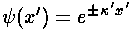where:'2=U0'-E'

Our matching points are x'=±½ (i.e., xa). The wavefunction and its derivative must be continuous at the matching point. The second derivative ofwill have a step at xa to match the step in potential as required by Schrödinger's equation: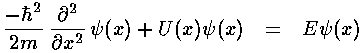Thus we require: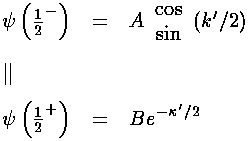Here the expression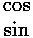indicates that we are to use the top item (cos) for odd n and the bottom item (sin) for even n. The expression: ½- indicates evaluation a little less ("-") than ½ i.e., in the classically allowed region and ½+ indicates evaluation a little more ("+") than ½, i.e., in the classically disallowed region. (Technically what's going on here is limits from the left hand side and limits from the right hand side. We are making sure that our formulae foragree at the turning point.) A and B are unknown constants having to do with the normalization of the wavefunction in each region.

Now we match the derivative of:We can eliminate the unknowns A and B by dividing the second equation by the first.

For odd n we have: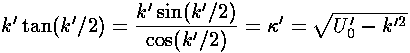For even n we have:The quantity formed by dividing the derivative ofby:is known as the logarithmic derivative since it is the derivative of ln(). Thus we would say the above two expressions require the continuity of the logarithmic derivative across the turning points.

The above two equations are nonlinear equations we can solve for k', and from k' we can find E'. The solutions, of course, depend on U0'. Once we know k', we can solve the-match equations for A/B. The overall scale ofis set by the normalization requirement:We are seeking the energy levels for various possible values of U0'. Here are the results for U0'=120:The match, so carefully made in the above work, is hard to see. In the below we show the n=4 wavefunction and blow up one matching region. Notice that on the left of x'=½ the curvature (second derivative) ofis positive whereas on the right of x'=½ the curvature is smaller and negative. The jump in curvature is required by Schrödinger's equation. A line with the matching slope is shown in blue.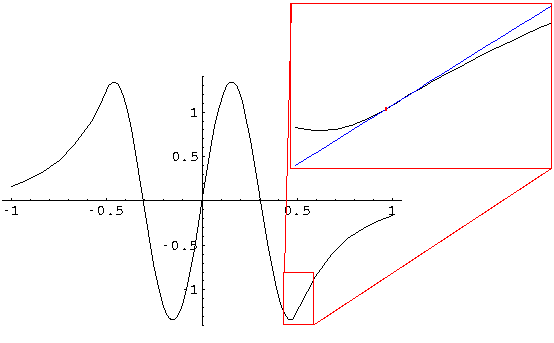Here are the results for U0'=100:If we reduce U0' to 80, the n=4 state is lost:Notice that as U0' is reduced, all the states move a bit lower in energy. For example, the third state was at E'=61.3 for U0'=120 and moved to 55.6 for U0'=80. With U0'=, third state has E'=(3)2=88.8. Note how the probability of being found in the classically disallowed region grows as E' nears U0'. The smaller the energy "deficit" in the classically disallowed region, the more likely it is to find a particle in this "disallowed" region. As we said earlier, if U0'=the wavefunction must be exactly zero in the disallowed region.

In the below plot we display the loss of states as U0' is reduced from infinity (i.e., 1/U0' increases from 0). On the y-axis k'/is plotted; Recall: E'=k'2. For the infinite square well k'=n, so for 1/U0'=0 the nth level's k'/is n and and states exist for each whole number n. As U0' is reduced each level's k' is reduced until k'/=n-1 at which point that level disappears. Thus for U0'=100 (1/U0'=.01) we have but 4 levels left, and the fourth is about to disappear.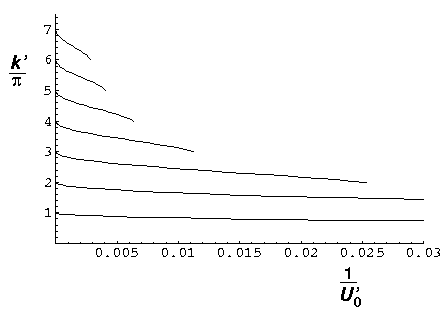The n=2 level disappears at U0'=2 (near 1/U0'=0.1), the ground state remains for even arbitrarily small U0'.